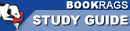Study Guide

# The Logic of Scientific Discovery - Part II: Chapter 9, Some Observations on Quantum Theory Summary & Analysis

This Study Guide consists of approximately 34 pages of chapter summaries, quotes, character analysis, themes, and more - everything you need to sharpen your knowledge of The Logic of Scientific Discovery.
 This section contains 618 words (approx. 2 pages at 400 words per page) View a FREE sample## Part II: Chapter 9, Some Observations on Quantum Theory Summary and Analysis

In this chapter, Popper tests his probability theory on some basic questions in quantum physics. Popper cites quantum physics as one of the premier scientific achievements so doing this is a true test of his own theories. Taking on Heisenberg's Uncertainty Principle, he seeks to show how it contains probability statements that must be interpreted statistically to be useful. He also suggests that some of Heisenberg's assumptions actually contradict quantum principles if they are statistically interpreted. In this exercise, he uses no mathematical arguments and only a single mathematical theorem.

Heisenberg's attempt to eliminate unobservable events to answer criticisms of Bohr's work with the electron is compared to Einstein's issues of hiding unobservable events. At the atomic level, the energy exchange between an object and the measuring...

(read more from the Part II: Chapter 9, Some Observations on Quantum Theory Summary)

 This section contains 618 words (approx. 2 pages at 400 words per page) View a FREE sampleMore summaries and resources for teaching or studying The Logic of Scientific Discovery.